Next: Examples of Applications Up: Track Fitting in r-z Previous: Pivot Transformation

## Track Fitting with Multiple Scattering

Now let us turn our attention to the r-z track fitting under the influence of multiple scattering. The treatment here provides a way to calculate the E0 of the last subsection including multiple scattering effects in track fitting. From now on, we take (y0,z0) = (0,0) and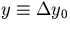.The multiple scattering modifies the track parameter of the particle as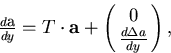(90)
where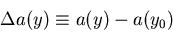(91)
represents the change of the dip angle due to the multiple scattering as a function of y. This results in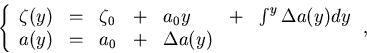(92)
where the parmeters with the suffix are those at the initial point before multiple scattering.

Now, let us assume that we are given (n+1) hit points,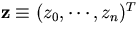,measured at fixed y positions,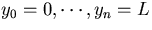.Then the minimization of the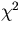: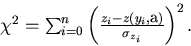(93)
The parameter vector that gives theminimum is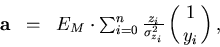(94)
with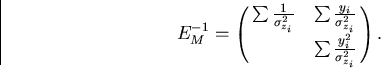(95)
Through the above equation, the change in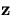due to the multiple scattering induces a change in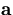: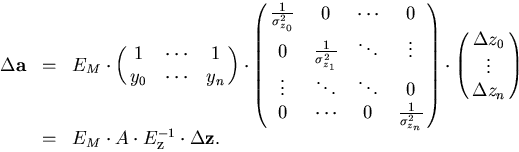(96)
From this we obtain the full error matrix including multiple scattering: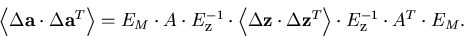(97)

Again the problem reduces to the evaluation of the correlation matrix in the coordinate measurements: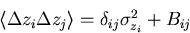(98)
with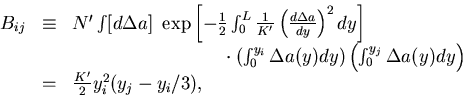(99)
where the last expression is valid for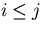.The full error matrix is then written in the form: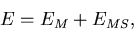(100)
where the first term is from the coordinate measurement errors while the second term from the multiple scattering: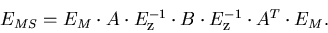(101)

In the case of equal space sampling with the same position resolution, EM and EMS reduce to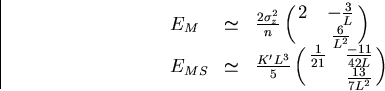(102)
with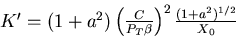(103)
in the large n limit.Next: Examples of Applications Up: Track Fitting in r-z Previous: Pivot Transformation
Keisuke Fujii
12/4/1998